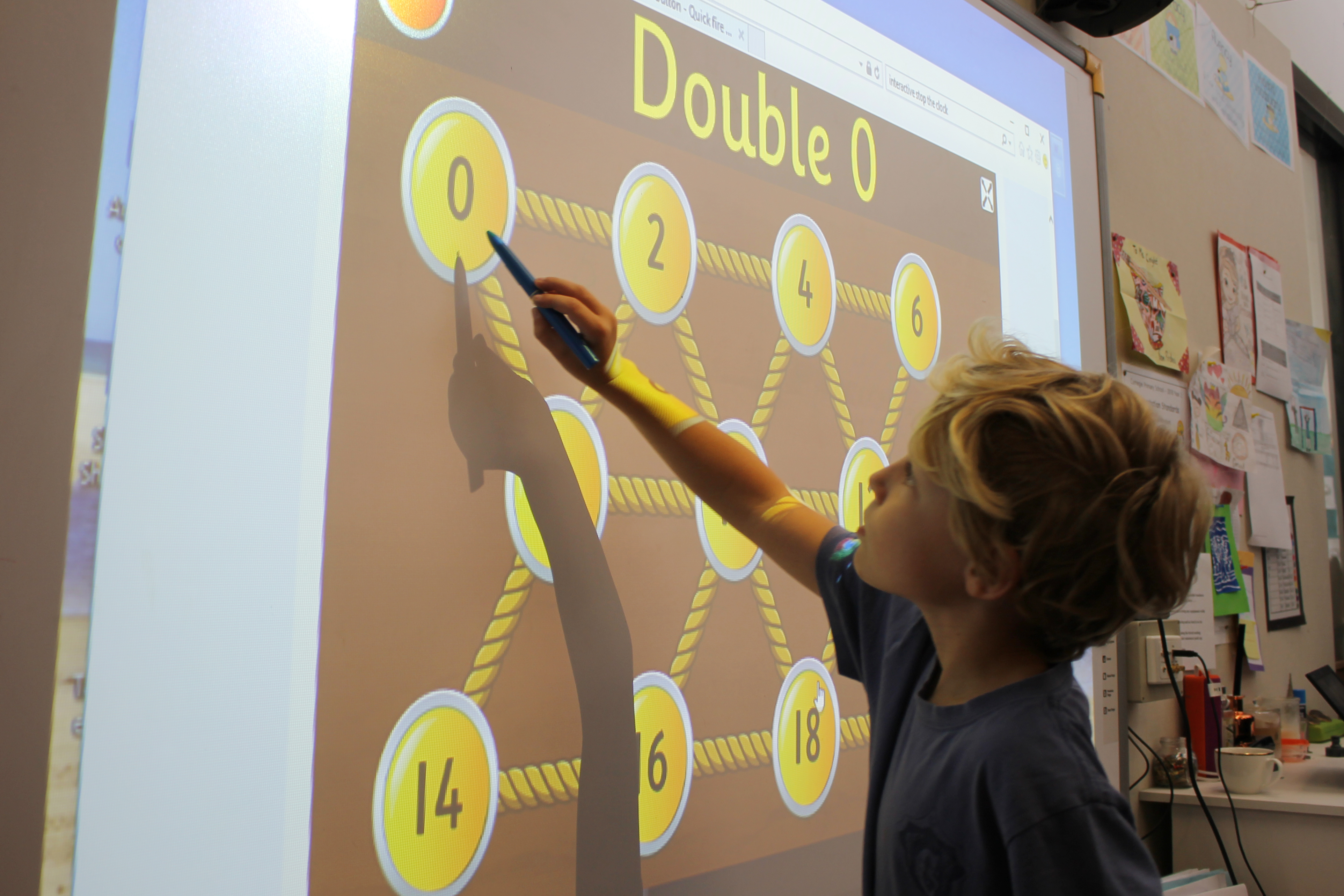### Numeracy

Our mathematics program provides opportunities for all students to become confident, mathematical problem solvers and to learn to communicate and reason mathematically.

We aim to provide mathematical learning for all students by setting attainable objectives for each child. By implementing a sequentially developed program based on previously acquired concepts and skills where authentic links can be made to our Discovery Units and real life situations. This will further support the child’s understanding.The general structure of the mathematics program is divided into three main dimensions:

Number and Algebra
Number and algebra are developed together. Students apply number sense and strategies for counting, representing numbers and computation. They recognise patterns, understand connections between operations, describe number relationships and formulate generalisations. Number and algebra skills are used to conduct investigations, solve problems and communicate reasoning.

Measurement and Geometry
The practical relationship between measurement and geometry is emphasised. Students study size, shape, relative position and movement of two-dimensional and three-dimensional objects in space. They measure quantities using appropriate metric units, and build understanding of measures such as length, perimeter, area, volume, capacity and mass.

Statistics and Probability
Students recognise and analyse data and draw inferences. They represent, summarise and interpret data. They assess likelihood and assign probabilities.